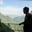Related Tags

fortran
array
dynamic
allocate
communitycreator

# What are dynamic arrays in Fortran?AKASH BAJWA

### Dynamic arrays

An array whose size is not defined at the compilation time but is defined at run time is known as a dynamic array.

For the declaration of this array, we use the keyword allocatable. These arrays are quite helpful to accurately use the required memory at the required time.

### Syntax

Let’s discuss the basic syntax of dynamic arrays.

#### One-dimensional

type, dimension(: ), allocatable :: name


#### Two-dimensional

type, dimension(:,: ), allocatable :: name


#### n-dimensional

To create n-dimensional arrays, repeat : $n$ times in the dimension() method.

### One-dimensional dynamic arrays

After defining the dimension of an array, we have to use the allocate() function to allocate the memory to the defined array.

Allocate(arr1(v1))


We can also use the deallocate() function to free the memory location.

Deallocate(arr1)


Integer, dimension ( : ), Allocatable :: arr1

Allocate(arr1(v1))

Deallocate(arr1)

### Two/n-dimensional dynamic arrays

To initialize a two-dimensional array, use the code below.

Integer, dimension ( : , : ), Allocatable :: arr2


To allocate the memory space:

Allocate(arr2(v1 , v2))


To deallocate:

Deallocate(arr2)


Integer, dimension ( : , : ), Allocatable :: arr2

Allocate(arr2(v1 , v2))

Deallocate(arr2)


### Code

The code snippet below demonstrates the use of dynamic arrays.

# While making exercises "package Test;" needs to be the first line of code.
program example_da
implicit none
real, dimension (:,:), allocatable ::  arr1
integer :: v1, v2
allocate ( arr1(v1,v2) )
arr1(v1,v2) = v1 * v2
print*, "arr1(",v1,",",v2,") = ", arr1(v1,v2)
deallocate (arr1)
end program example_da

RELATED TAGS

fortran
array
dynamic
allocate
communitycreator

CONTRIBUTORAKASH BAJWA
RELATED COURSES

View all Courses

Keep Exploring

Learn in-demand tech skills in half the time